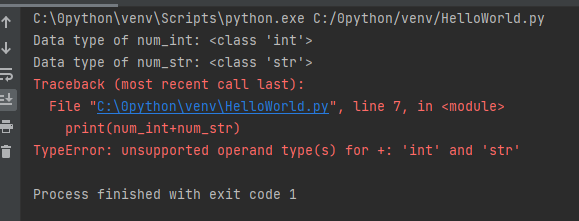# Python 类型转换和强制类型转换

• 隐式类型转换
• 显式类型转换

## 2.隐式类型转换

``````num_int = 123
num_flo = 1.23

num_new = num_int + num_flo

print("datatype of num_int:",type(num_int))
print("datatype of num_flo:",type(num_flo))

print("Value of num_new:",num_new)
print("datatype of num_new:",type(num_new))``````

``````datatype of num_int: <class 'int'>
datatype of num_flo: <class 'float'>
Value of num_new: 124.23
datatype of num_new: <class 'float'>

Process finished with exit code 0``````

• 整型变量 num_int 和浮点变量 num_flo相加，将值存储在 num_new 中；
• 程序分别查看所有三个对象的数据类型；
• 在输出中，我们可以看到 num_int 的数据类型是整数，而 num_flo 的数据类型是浮点数；
• 输出结果可以看到 num_new 具有浮点数据类型，因为 Python 总是将较小的数据类型转换为较大的数据类型，以避免数据丢失。

``````num_int = 123
num_str = "456"

print("Data type of num_int:",type(num_int))
print("Data type of num_str:",type(num_str))

print(num_int+num_str)``````• 两个变量 num_int （整型）和 num_str （字符串）相加。
• 从输出中可以看出，我们得到了 TypeError。 Python 在这种情况下无法使用隐式转换。
• 但是，Python 为这些类型的情况提供了一种解决方案，称为显式转换。

## 3.显式类型转换

``<required_datatype>(expression)``

``````num_int = 123
num_str = "456"

print("Data type of num_int:",type(num_int))
print("Data type of num_str before Type Casting:",type(num_str))

num_str = int(num_str)
print("Data type of num_str after Type Casting:",type(num_str))

num_sum = num_int + num_str

print("Sum of num_int and num_str:",num_sum)
print("Data type of the sum:",type(num_sum))
``````

``````Data type of num_int: <class 'int'>
Data type of num_str before Type Casting: <class 'str'>
Data type of num_str after Type Casting: <class 'int'>
Sum of num_int and num_str: 579
Data type of the sum: <class 'int'>

Process finished with exit code 0``````

• 两个变量 num_str （字符串型）和 num_int （整数型）相加。
• 我们使用 int() 函数将 num_str 从 字符串（高）转换为 整数型（低） 类型以执行加法。
• 将 num_str 转换为整数值后，Python 可以将这两个变量相加。
• 程序自动将 num_sum 数据类型设为整数。

## 关键点

• 类型转换是将对象从一种数据类型转换为另一种数据类型。
• 隐式类型转换由 Python 解释器自动执行。
• Python 避免了隐式类型转换中的数据丢失。
• 显式类型转换也称为强制类型转换，对象的数据类型由用户使用预定义的函数进行转换。
• 在类型铸造（强制类型转换）中，当我们将对象强制为特定数据类型时，可能会发生数据丢失。

wer

(Visited 156 times, 2 visits today)

Posted in Python教程